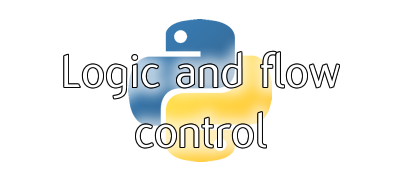# Python for GCSE

2: Logic and flow control

Revision tools
You can print this page for a quick reference guide or you can use the tools below to create printable test sheets
2.1: understand the selection statement and nested selectionKeywordDefinition
indentationadding tabs or spaces to the start of a line to affect when it should be run
nestedwhen an instruction is indented so that it runs within another section of code (e.g. if statement within another if statement)
programming constructprogramming building block e.g sequence, selection or iteration
selectionprogramming construct used whenever a program has to make a decision (e.g. if statement)
2.2: be able to use: equal to; not equal to; less than; greater than; less than or equal to; greater than or equal toKeywordDefinition
!=check if two values are not equal
<True if the value on the left is less than the value on the right
<=True if the value on the left is less than or equal to the value on the right
==True if two values are equal
>True if the value on the left is greater than the value on the right
>=True if the value on the left is greater than or equal to the value on the right
comparative operatorsymbol used to compare
2.3: be able to use and understand AND, OR, NOT and XOR logical operators as symbols and in selection criteriaKeywordDefinition
andlogical operator which combines two boolean expressions and results in True if both inputs are True
boolean expressionquestion which has an answer of either True or False
logical operatorkeyword which allows you to combine boolean expressions into a single boolean value
notlogical operator which gets the opposite boolean value of a single input
orlogical operator which combines two boolean expressions and results in True if either or both inputs are True
xorlogical operator which combines to boolean expressions and results in True if only one of the inputs is True